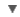POS
HYPHEN
WORDNET DICTIONARY
CIDE DICTIONARY
OXFORD DICTIONARY
Link, Gadget and Shareordinate

:
:
or=di=nate

CIDE DICTIONARY

ordinatea. [L. ordinatus, p. p. of ordinare. See Ordain.].
Well-ordered; orderly; regular; methodical.  Chaucer.  [1913 Webster]
Ordinate figure (Math.), a figure whose sides and angles are equal; a regular figure.
ordinaten.
The distance of any point in a curve or a straight line, measured on a line called the axis of ordinates or on a line parallel to it, from another line called the axis of abscissas, on which the corresponding abscissa of the point is measured.  [1913 Webster]
" The ordinate and abscissa, taken together, are called coördinates, and define the position of the point with reference to the two axes named, the intersection of which is called the origin of coördinates. In a typical two-dimensional plot, viewed on a plane graph in its normal orientation with perpendicular axes, the ordinate is the vertical axis; when the axes are labeled as x and y, it is the y-axis. See Coordinate."  [1913 Webster]
ordinatev. t.
To appoint, to regulate; to harmonize.  Bp. Hall.  [1913 Webster]

OXFORD DICTIONARY

ordinate, n. Math. a straight line from any point drawn parallel to one coordinate axis and meeting the other, usually a coordinate measured parallel to the vertical (cf. ABSCISSA).

Etymology
L linea ordinata applicata line applied parallel f. ordinare: see ORDAIN
copyright © 2012 Yayasan Lembaga SABDA (YLSA) | To report a problem/suggestion